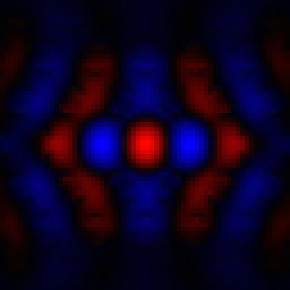OpenCL 3D electromagnetic FDFD solver
You can not select more than 25 topics Topics must start with a letter or number, can include dashes ('-') and can be up to 35 characters long.

#### 65 lines 2.3 KiB Raw Permalink Normal View History Unescape Escape6 years ago ```# opencl_fdfd ``` ``` ```6 years ago ```**opencl_fdfd** is a 3D Finite Difference Frequency Domain (FDFD) ```6 years ago ```electromagnetic solver implemented in Python and OpenCL. ```6 years ago ``` ``` ``` ``` ```**Capabilities:** ```6 years ago ```* Arbitrary distributions of the following: ```2 years ago ``` * Dielectric constant (`epsilon`) ``` ``` * Magnetic permeabilty (`mu`) ``` ``` * Perfect electric conductor (`PEC`) ``` ``` * Perfect magnetic conductor (`PMC`) ```6 years ago ```* Variable-sized rectangular grids ``` ``` * Stretched-coordinate PMLs (complex cell sizes allowed) ``` ``` ``` ```Currently, only periodic boundary conditions are included. ``` ```PEC/PMC boundaries can be implemented by drawing PEC/PMC cells near the edges. ``` ```Bloch boundary conditions are not included but wouldn't be very hard to add. ``` ``` ```2 years ago ```The default solver `opencl_fdfd.cg_solver(...)` located in main.py ```6 years ago ```implements the E-field wave operator directly (ie, as a list of OpenCL ``` ```instructions rather than a matrix). Additionally, there is a slower ```2 years ago ```(and slightly more versatile) solver in `csr.py` which attempts to solve ```6 years ago ```an arbitrary sparse matrix in compressed sparse row (CSR) format using ``` ```the same conjugate gradient method as the default solver. The CSR solver ``` ```is significantly slower, but can be very useful for testing alternative ``` ```formulations of the FDFD electromagnetic wave equation. ```6 years ago ``` ``` ```Currently, this solver only uses a single GPU or other OpenCL accelerator; ``` ```generalization to multiple GPUs should be pretty straightforward ``` ```(ie, just copy over edge values during the matrix multiplication step). ``` ``` ``` ``` ```6 years ago ```## Installation ``` ``` ```6 years ago ```**Dependencies:** ```2 years ago ```* python 3 (written and tested with 3.7) ```6 years ago ```* numpy ``` ```* pyopencl ``` ```* jinja2 ```2 years ago ```* [meanas](https://mpxd.net/code/jan/meanas) (>=0.5) ```6 years ago ``` ``` ``` ``` ```Install with pip, via git: ``` ``````bash ```4 years ago ```pip install git+https://mpxd.net/code/jan/opencl_fdfd.git@release ```6 years ago `````` ``` ``` ``` ``` ``` ```## Use ``` ``` ```2 years ago ```See the documentation for `opencl_fdfd.cg_solver(...)` ```6 years ago ```(located in ```main.py```) for details about how to call the solver. ```6 years ago ```The FDFD arguments are identical to those in ```2 years ago ````meanas.solvers.generic(...)`, and a few solver-specific ```6 years ago ```arguments are available. ```11 months ago ``` ```6 years ago ```An alternate (slower) FDFD solver and a general gpu-based sparse matrix ```2 years ago ```solver is available in `csr.py`. These aren't particularly ```6 years ago ```well-optimized, and something like ``` ```[MAGMA](http://icl.cs.utk.edu/magma/index.html) would probably be a ``` ```better choice if you absolutely need to solve arbitrary sparse matrices ```6 years ago ```and can tolerate writing and compiling C/C++ code. Still, they're ``` ```usually quite a bit faster than the scipy.linalg solvers. ```